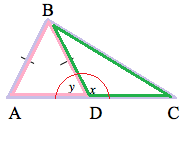### Home > MC2 > Chapter 8 > Lesson 8.2.5 > Problem8-80

8-80.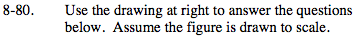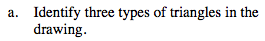The three triangles are highlighted:

Triangle ABD has two sides of equal length.
Notice the size of the angles of the other two triangles.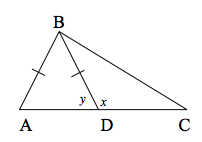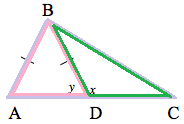Triangle ABC

Triangle ABD

Triangle BCD

Triangle ABD is an isosceles triangle, triangle ABC is acute, and triangle BCD is obtuse.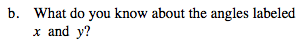If two angles are supplementary, what is their sum?